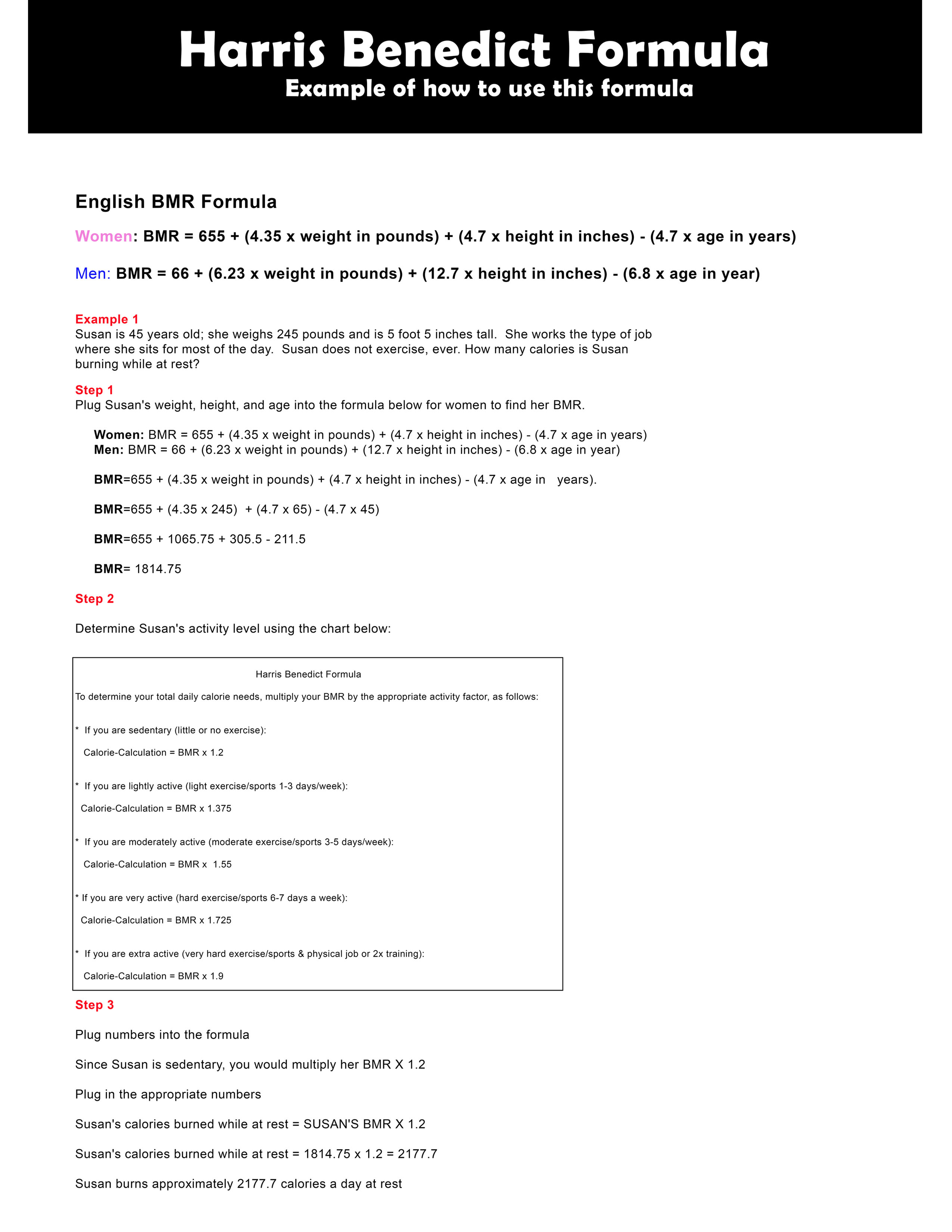# Harris Benedict Formula Example

Here's a sample showing you how to use the Harris Benedict Equation to determine the amount of calories you burn while resting.

WARNING: Use this formula only as an estimate.Download Medium size (17" by 11") Harris Benedict formula chart.

Example 1

Susan is 45 years old; she weighs 245 pounds and is 5 foot 5 inches tall.  She works the type of job where she sits for most of the day.  Susan does not exercise, ever. How many calories is Susan burning while at rest?

Step 1

Plug Susan's weight, height, and age into the formula below for women to find her BMR.

 English BMR Formula Women: BMR = 655 + (4.35 x weight in pounds) + (4.7 x height in inches) - (4.7 x age in years)Men: BMR = 66 + (6.23 x weight in pounds) + (12.7 x height in inches) - (6.8 x age in year)

BMR=655 + (4.35 x weight in pounds) + (4.7 x height in inches) - (4.7 x age in   years).

BMR=655 + (4.35 x 245)  + (4.7 x 65) - (4.7 x 45)

BMR=655 + 1065.75 + 305.5 - 211.5

BMR= 1814.75

Step 2

Determine Susan's activity level using the chart below

 Harris Benedict Formula To determine your total daily calorie needs, multiply your BMR by the appropriate activity factor, as follows:   *  If you are sedentary (little or no exercise): Calorie-Calculation = BMR x 1.2   *  If you are lightly active (light exercise/sports 1-3 days/week): Calorie-Calculation = BMR x 1.375   *  If you are moderately active (moderate exercise/sports 3-5 days/week): Calorie-Calculation = BMR x  1.55   * If you are very active (hard exercise/sports 6-7 days a week): Calorie-Calculation = BMR x 1.725   *  If you are extra active (very hard exercise/sports & physical job or 2x training): Calorie-Calculation = BMR x 1.9

Step 3

Plug numbers into the formula

Since Susan is sedentary, you would multiply her BMR X 1.2

Plug in the appropriate numbers

Susan's calories burned while at rest = SUSAN'S BMR X 1.2

Susan's calories burned while at rest = 1814.75 x 1.2 = 2177.7

Susan burns approximately 2177.7 calories a day at rest

Example 2

If Susan decided to exercise and burn 600 calories how many TOTAL calories would she burn?

Step 1

Plug numbers into the formula below:

Susan's calories burned resting + amount of calories burned exercising.

Susan burns 2177.7 calories resting. If she burns an additional 600 calories exercising Susan would burn 2177.7+600=2877.7

Susan's total calories burned is 2877.7

If Susan wants to shed excess weight for that day she would have to eat less than 2877.7 calories.